# The Inner Number Patterns – 4/7

### Number patterns through the Cube

Each of the number patterns 1 to 8 rotate ninety degrees from face to opposite face of the cube.

Each number pattern changes shape within the cube, but returns to its original shape on the opposite cube face, but rotating 90 degrees as mentioned above. However, each number pattern lies directly opposite its coupling number i.e. the number 1 pattern lies directly in line with the number 8 pattern on the opposite face, as does 2 with 7, 3 with 6 and 4 with 5.

#### Three dimensional images of the number cube and numbers within the number cube, all taken at a 45 degree viewpoint.

The number colours shown are as shown in the previous levels of the Ant Cube. The outer shell of nines is left off except for a part shell shown in the last image.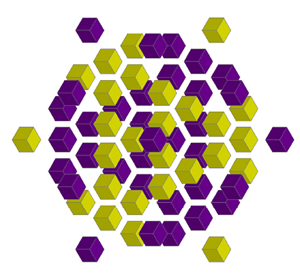Coupling Numbers 1 and 8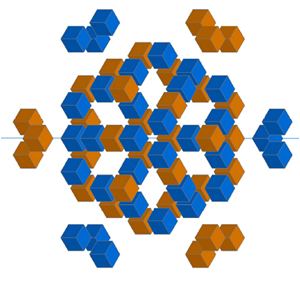Coupling Numbers 2 and 7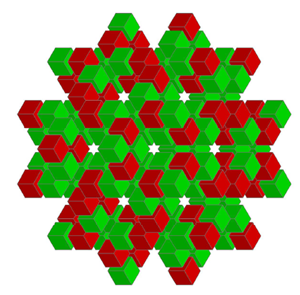Coupling Numbers 3 and 6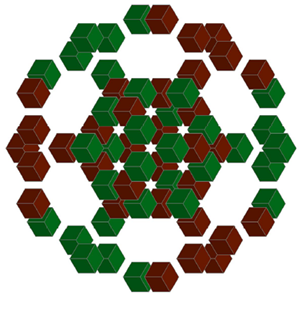Coupling Numbers 4 and 5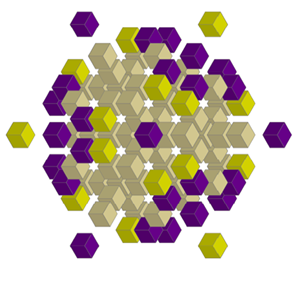Coupling Numbers 1, 8 with 9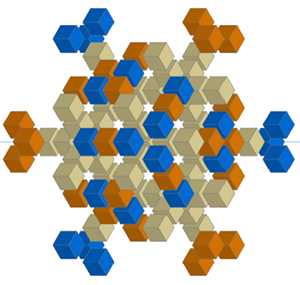Coupling Numbers 2, 7 with 9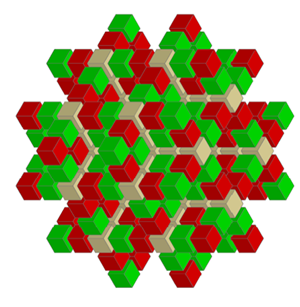Coupling Numbers 3, 6 with 9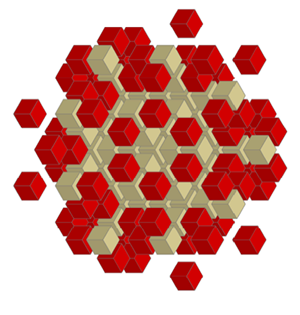Number 6 only with 9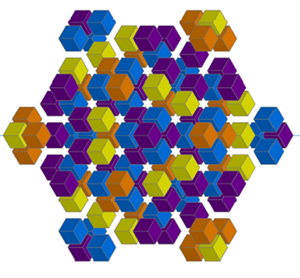Coupling Numbers 1+ 8, 2 + 7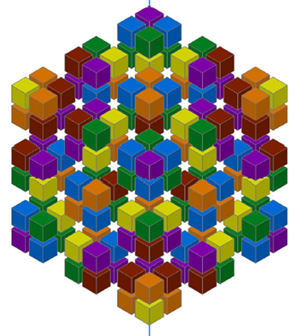Coupling Numbers 1 + 8, 2 + 7, 4 + 5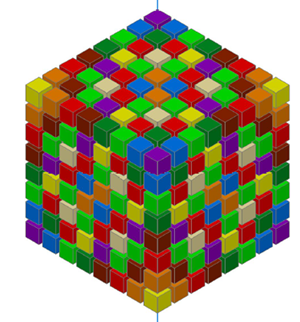All Coupling Numbers 1 + 8, 2 + 7, 3 + 6, 4 + 5 with 9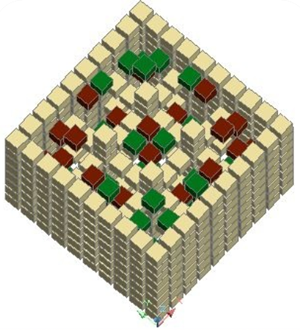4, 5 and 9 shell view in at 45 deg

Whilst switching on individual numbers and rotating the number Cube, I noticed something that I had not realised before. When looking square-on through the cube, from any side, each number occupies all the gaps between the number 9 ‘rods’ or framework as seen below, even though the number will sit in varied locations at different levels. Example:  numbers 4 and 1 shown below.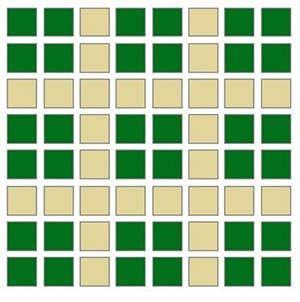Number 4 viewed through depth of Cube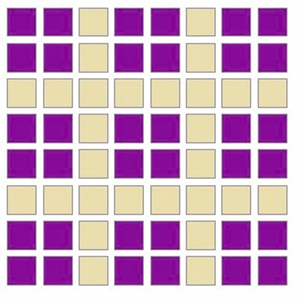Number 1 viewed through depth of Cube

After exploring many number sequence arrangements, there seems to me to be an almost limitless number of interesting mathematical links and interrelationships between the numbers, number sequences and number patterns. This discovery may help reveal underlying mathematics in number patterns and shapes in nature and possibly help in the understanding of the structural function of the universe. This sounds like a tall order for a relatively simple cube of numbers, but there is a great deal of seemingly simple complexity hidden within what I have discovered. I do not consider this cube of number patterns as an invention or idea, it is however an important discovery of the actual hidden potential already existing in the relationship of our numbers. I believe that this number cube will reveal many useful codes and facets once investigated in depth.

The significance of the number 9 as a ‘structural’ entity or form, fundamental to the mathematics of our world and possibly the universe, was to crystalise later in my life, as was the importance of the coupling numbers 1 with 8, 2 with 7, 3 with 6 and 4 with 5 in the strong link with very ancient sites. Ancient and ‘Sacred’ numbers passed down through time, i.e. in religious texts, legends, stories, writings and in structures such as the Pyramids of Giza, Stonehenge, Avebury, Angkor Wat, Teotihuacan, Ollantaytambo, Easter Island etc, portray or reveal great significance regarding the number 9 and links to the same coupling numbers revealed in my Cube. After the following section on ‘the missing number zero’ I will describe some of these links.

### Interesting math regarding the missing number zero (0)

I have pondered how and why the number 0 fits in so importantly with our system of numbering, especially the logic of decimal mathematics and arithmetic, and yet is not an integral part of this 3D numbering Cube. The number 0 represents nought or nothing, however it becomes a countable number when combined with the numbers 1 to 9 in our numbering system; for example after the number 9 (as the number 10), in other words after the first completed set of nine numbers. Even though not represented as a resultant number on the cube, the zero allows the number cube to work as it does:-  For example 2 x 5 = 10, 1 + 0 = 1. Without the involvement of the zero the numbering of neither the 2D Square nor 3D Cube would work to create the number patterns. Note that the number 11 is to be added as 1 + 1 = 2, which correctly reflects that this is the second number, 2, after number 9 i.e. 9 + 1 + 1 = 11=2. The same applies to all the numbers; – the logic is that the number 0 is not actually ‘counted’ as a number but is a number 1 to 9 set group marker and latent facilitator for the addition, subtraction, or multiplication of any combination of the counted numbers 1 to 9. A few more examples will help clarify my point.

9 + 3 = 12. In the square or cube numbering system the number 12 must be brought back to a single number by further adding its integers, so 12 = 1 + 2 = 3. The number 12 is 3 numbers after 9 and results therefore in a 3.

18 + 5 = 23. The number 23 is the fifth number after the previous whole number multiple of 9 (which is 18). To clarify, in the square or cube numbering system the number 23 results in 2 + 3 = 5.

6 x 5 = 30. In the square or cube numbering system the number 30 has its integers added to obtain the single number 3. Also the number 30 is the 3rd number after the previous whole number multiple of 9, which is 27, i.e. 27 plus 3 = 30. 30 = 3 + 0 = 3.